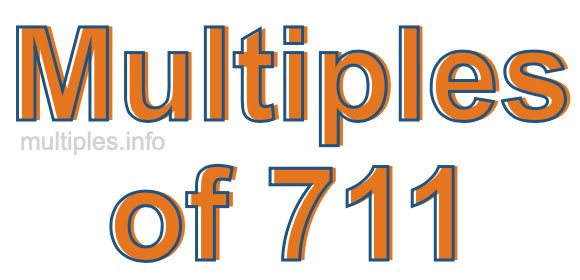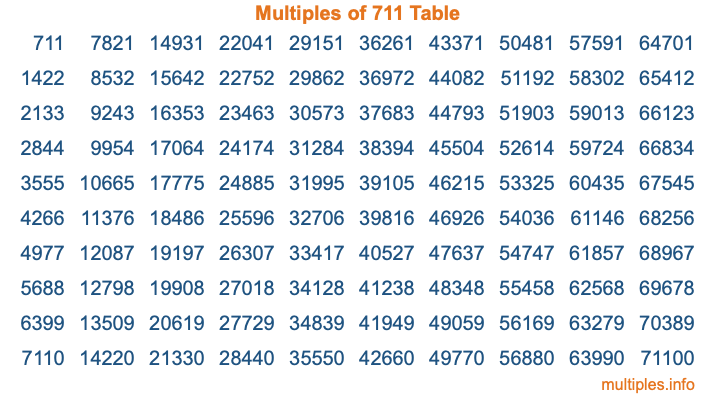Multiples of 711Welcome to the Multiples of 711 page. Here we will first teach you everything you will ever need to know about the multiples of 711, and then give you a study guide summary of everything we taught you to make sure you remember it all. Use this page to look up facts and learn information about the multiples of 711. This page will make you a multiples of seven hundred eleven expert!

Definition of Multiples of 711
Multiples of 711 are all the numbers that when divided by 711 equal an integer. Each of the multiples of 711 are called a multiple. A multiple of 711 is created by multiplying 711 by an integer.

Therefore, to create a list of multiples of 711, you start with 1 multiplied by 711, then 2 multiplied by 711, then 3 multiplied by 711, and so on for as long as you want. Thus, the list of the first five multiples of 711 is 711, 1422, 2133, 2844, and 3555. To see a larger list of multiples of 711, see the printable image of Multiples of 711 further down on this page. We also have a category where you can choose any nth multiple of 711.

Multiples of 711 Checker
The Multiples of 711 Checker below checks to see if any number of your choice is a multiple of 711. In other words, it checks to see if there is any number (integer) that when multiplied by 711 will equal your number. To do that, we divide your number by 711. If the the quotient is an integer, then your number is a multiple of 711.

Is  a multiple of 711?

Least Common Multiple of 711 and ...
A Least Common Multiple (LCM) is the lowest multiple that two or more numbers have in common. This is also called the smallest common multiple or lowest common multiple and is useful to know when you are adding our subtracting fractions. Enter one or more numbers below (711 is already entered) to find the LCM.

Check out our LCM Calculator if you need more details about the Least Common Multiple or if you need the LCM for different numbers for adding and subtraction fractions.

nth Multiple of 711
As we stated above, 711 is the first multiple of 711, 1422 is the second multiple of 711, 2133 is the third multiple of 711, and so on. Enter a number below to find the nth multiple of 711.

th multiple of 711

Multiples of 711 vs Factors of 711
711 is a multiple of 711 and a factor of 711, but that is where the similarities end. All postive multiples of 711 are 711 or greater than 711. All positive factors of 711 are 711 or less than 711.

Below is the beginning list of multiples of 711 and the factors of 711 so you can compare:

Multiples of 711: 711, 1422, 2133, 2844, 3555, etc.

Factors of 711: 1, 3, 9, 79, 237, 711

As you can see, the multiples of 711 are all the numbers that you can divide by 711 to get a whole number. The factors of 711, on the other hand, are all the whole numbers that you can multiply by another whole number to get 711.

It's also interesting to note that if a number (x) is a factor of 711, then 711 will also be a multiple of that number (x).

Multiples of 711 vs Divisors of 711
The divisors of 711 are all the integers that 711 can be divided by evenly. Below is a list of the divisors of 711.

Divisors of 711: 1, 3, 9, 79, 237, 711

The interesting thing to note here is that if you take any multiple of 711 and divide it by a divisor of 711, you will see that the quotient is an integer.

Multiples of 711 Table
Below is an image of the first 100 multiples of 711 in a table. The table is in chronological order, column by column. The first column has the first ten multiples of 711, the second column has the next ten multiples of 711, and so on.The Multiples of 711 Table is also referred to as the 711 Times Table or Times Table of 711. You are welcome to print out our table for your studies.

Negative Multiples of 711
Although not often discussed or needed in math, it is worth mentioning that you can make a list of negative multiples of 711 by multiplying 711 by -1, then by -2, then by -3, and so on, to get the following list of negative multiples of 711:

-711, -1422, -2133, -2844, -3555, etc.

Multiples of 711 Summary
Below is a summary of important Multiples of 711 facts that we have discussed on this page. To retain the knowledge on this page, we recommend that you read through the summary and explain to yourself or a study partner why they hold true.

There are an infinite number of multiples of 711.

A multiple of 711 divided by 711 will equal a whole number.

711 divided by a factor of 711 equals a divisor of 711.

The nth multiple of 711 is n times 711.

The largest factor of 711 is equal to the first positive multiple of 711.

711 is a multiple of every factor of 711.

711 is a multiple of 711.

A multiple of 711 divided by a divisor of 711 equals an integer.

711 divided by a divisor of 711 equals a factor of 711.

Any integer times 711 will equal a multiple of 711.

Multiples of a Number
Here you can get the multiples of another number, all with the same attention to detail as we did for multiples of 711 on this page.

Multiples of
Multiples of 712
Did you find our page about multiples of seven hundred eleven educational? Do you want more knowledge? Check out the multiples of the next number on our list!